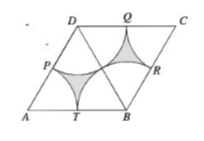# Triangles say it all

Geometry Level 2Let be $\triangle ABD$ and $\triangle BCD$ equilateral triangles of side $10$. $Q,R,S$ and $T$ are middle points of $\triangle ABD$ and (\triangle BCD.

Find the shaded area.

×

Problem Loading...

Note Loading...

Set Loading...